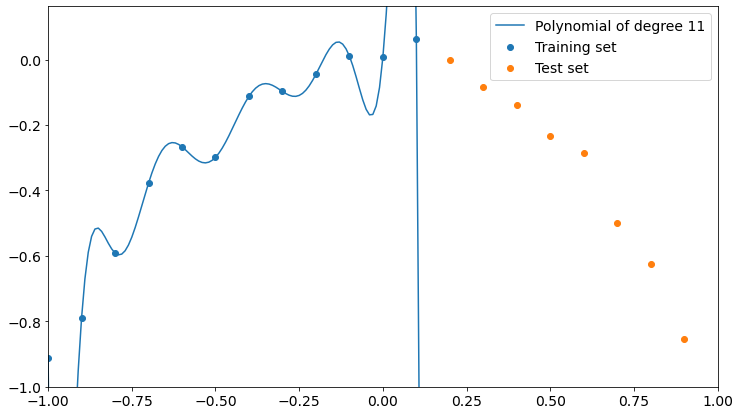# An example of overfitting and how to avoid it

Original article was published on Artificial Intelligence on Medium# What is overfitting?

Overfitting occurs when your model learns too much from training data and isn’t able to generalize the underlying information. When this happens, the model is able to describe training data very accurately but loses precision on every dataset it has not been trained on. This is completely bad because we want our model to be reasonably good on data that it has never seen before.

# Why does it happen?

In machine learning, simplicity is the key. We want to generalize the information obtained from the training dataset, so we can surely say that we run the risk of overfitting if we use complex models.

Complex models will likely over-learn from training data and will think that the random error that drifts training data from the underlying dynamics is actually worth learning from. That’s the exact point at which the model stops generalizing and starts overfitting.

Complexity is often measured with the number of parameters used by your model during it’s learning procedure. For example, the number of parameters in linear regression, the number of neurons in a neural network, and so on.

So, the lower the number of the parameters, the higher the simplicity and, reasonably, the lower the risk of overfitting.

# An example of overfitting

Let’s make a simple example with the help of some Python code.

I’m going to create a set of 20 points that follow the formula: